# Editing [Polygons]¶

Description: Sets the values of geographic data within the area of the Polygon. Figure 218 shows an example of the [Polygon].

## [Polygons Attribute Browser]¶

When [Polygons] data is selected in the [Object Browser], [Polygons Attribute Browser] is shown. Figure 219 shows an example of [Polygons Attribute Browser]. The list of columns in [Polygons Attribute Browser] is shown in Table 20 .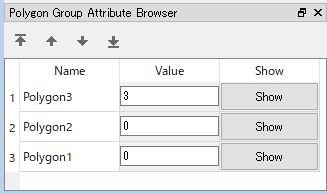Figure 219 Example of [Polygons Attribute Browser]

Table 20 The list of columns in [Polygons Attribute Browser]
Column name Description
Name The name of polygons. You can edit the values.
Value the valud of polygons. You can edit the values.
Show When clicked, the clicked polygon is shown in the center of the drawing area.

## Selecting operation¶

For [Polygons], user can select multiple polygons at the same time. the selected polygons can be deleted or sorted with one operation.

User can select polygons with the two ways below:

• Mouse operation: Draw boundary box by left-dragging, and all polygons sorrounded by the box are selected.
• Attribute browser operation: Click on items in [Polygons Attribute Browser], and the clicked item is selected. You can select multiple items, by clicking with pressing Ctrl key.

## [Add New Polygons]¶

The procedure to add a new [Polygons] is as follows:

1. In [Object Browser] ,select the group of [Geographic data] to which you want to add [Polygons] data (Figure 220) Please note that the list of groups of [Geographic Data] differs depending on the solver you are using.
2. Do the following operation. Then, a new [Polygons] data is added and selected in the object browser.

Menu bar: Geographic Data (E) –> Polygons (P) –> Add New Polygons

1. In the drawing area, define the nodes of the polygon, by left-clicking. (Figure 221)
2. Finish defining the shape of polygon, by double-clicking or pressing Enter key. Then the dialog to specify the value at the defined polygon is shown (Figure 222), so input the value and click on [OK]. Please note that the widget to edit value differs depending on the type of [Geographic Data].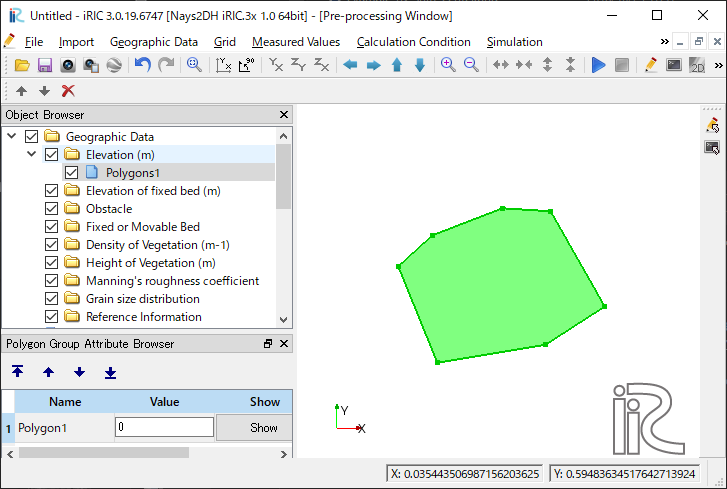Figure 221 Example of [Pre-processing Window] while defining a polygon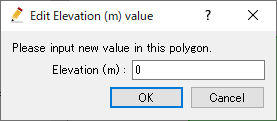Figure 222 Example of dialog to edit value of polygon

## [Add New Polygon]¶

Description: Adds a new Polygon to [Polygons] data.

[Add New Polygons] adds a new [Polygons] data. On the other hand, this function add an new Polygon to the [Polygons] data that already exists.

The steps to define a polygon is the same to [Add New Polygons].

## [Edit Value] (V)¶

Description: Edits data value within the Polygon.

When you select [Edit Value], the [Edit Elevation value] dialog (Figure 223) will open. Input a new value and click on [OK].

## [Add Vertex] (A)¶

Description: Adds a vertex to the [Polygon].

When you select [Add Vertex] and move the cursor to the edge of [Polygon], the cursor changes to that shown in Figure 224. Left click on the line and drag it to add a new vertex. The vertex is placed where you release the left button.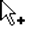Figure 224 Mouse cursor when possible to add a vertex

## [Remove Vertex] (R)¶

Description: Deletes a vertex from the [Polygon].

When you select [Remove Vertex] and move the cursor onto the vertex you want to remove, the cursor changes to that shown in Figure 225. Left clicking will remove the vertex.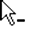Figure 225 Mouse cursor when possible to removing the vertex

## [Edit Coordinates] (C)¶

Description: Edits the coordinates of the vertices of the [Polygon].

When you select [Edit Coordinates], the [Polygon Coordinates] dialog (Figure 226) will open. Edit the coordinates and click on [OK].

## [Add Hole Region] (H)¶

Description: Adds the Hole Region to the [Polygon].

1. When you select [Add Hole Region], the [Information] dialog (Figure 227) will open. Click on [OK].
2. On the canvas, add vertexes to the Hole Region by left clicking (Figure 228).
3. Double click or press the Enter key to complete defining the Hole Region.

## [Delete Hole Region] (D)¶

Description: Deletes the Hole Region from the [Polygon].

1. When you select [Delete Hole Region], the [Warning] dialog (Figure 229) will open. Click on [Yes].
2. On the canvas, the Hole Region will be deleted. (Figure 230).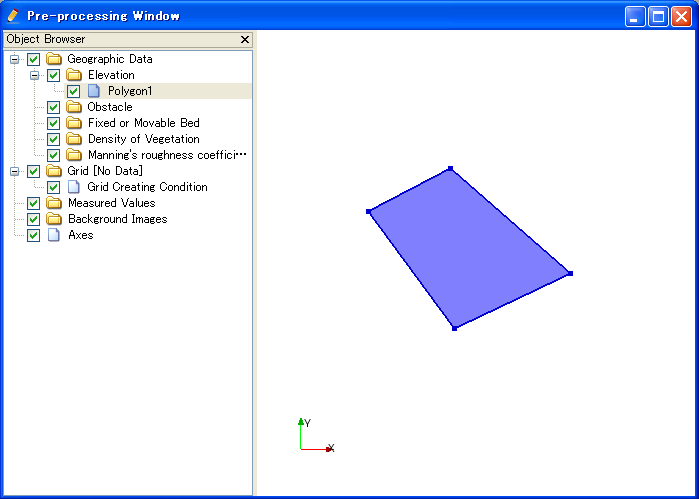Figure 230 Example of the [Delete Hole Region] Result

## [Merge]¶

Description: Merge polygons in other [Polygons] to this data.

[Select data to merge] dialog (Figure 231) is shown. Select the data to merge, and click on [OK] button.

## [Copy]¶

Description: Copy the data to other [Geographic Data] group.

[Select Graographic Data] dialog (Figure 232) is shown. Select the [Geographic Data] group to which you want to copy the data.

Then, [Copy Setting] dialog (Figure 233) is shown. Specify the values of the polygons, and click on [OK] button, to finish copying the [Polygons] data.

## [Color Setting] (S)¶

Description: Edits the color of the [Polygon].

When you select [Color Setting], the [Polygon Color] dialog (Figure 234) will open. Set it and click on [OK].